# 如何由计算解实现傅里叶变换

2017年 2月 27日

### 用傅里叶光学进行傅里叶变换

E(u,v,f) = \frac{-1}
{i\lambda f}
e^{i2\pi f /\lambda} e^{-i\pi(u^2+v^2)/(\lambda f)} \iint_{\infty}^
{\infty}
E(x,y,0)e^{-i \pi(x^2+y^2)/(\lambda f)}e^
{i2 \pi (xu+yv)/(\lambda f)}
dxdy,

(+i\omega t)
。因此，二次相位函数的符号为负。

#### 菲涅尔透镜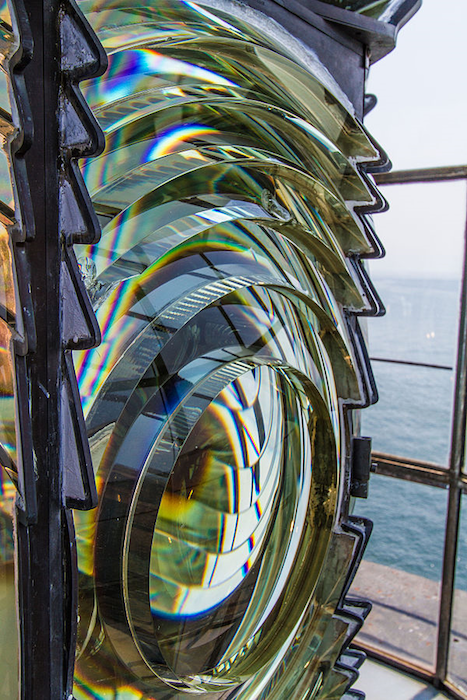### 在 COMSOL Multiphysics® 中模拟聚焦菲涅尔透镜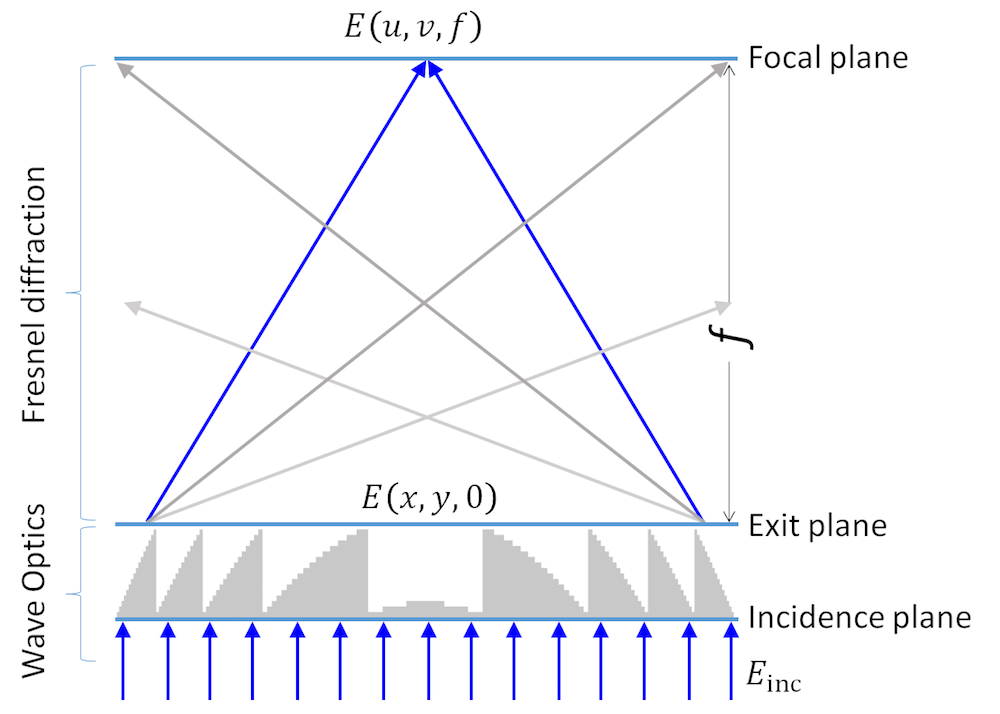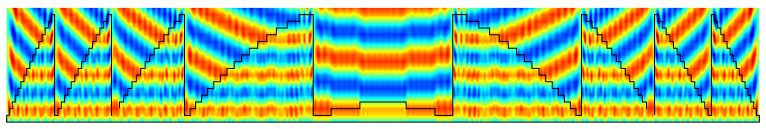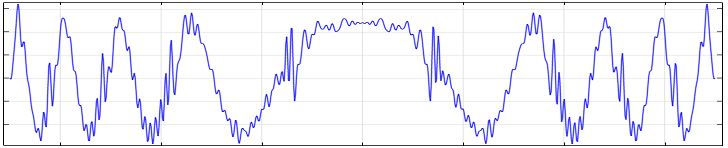### 通过计算解实现傅里叶变换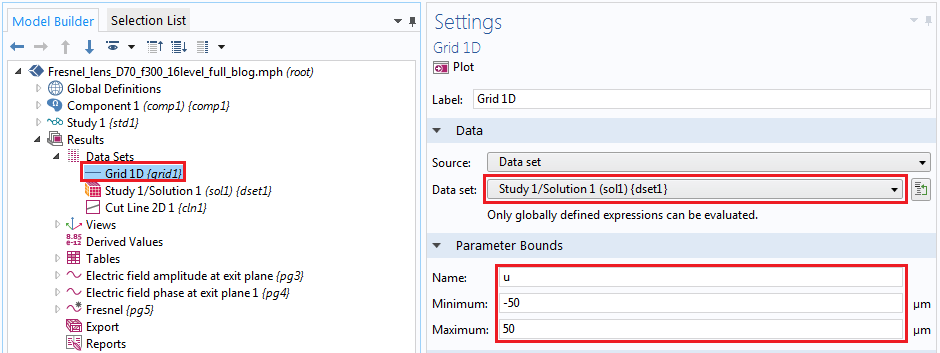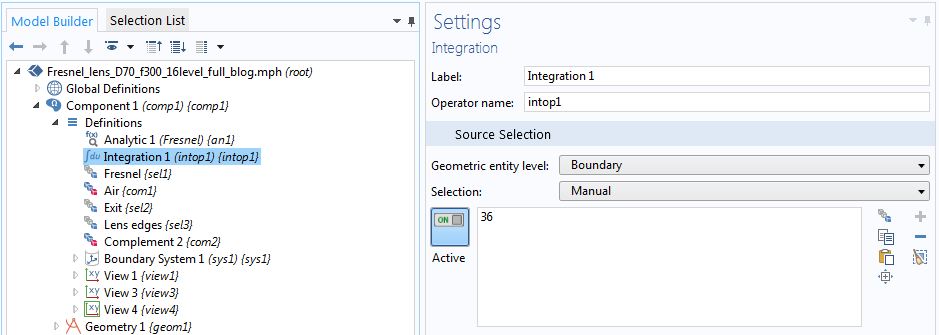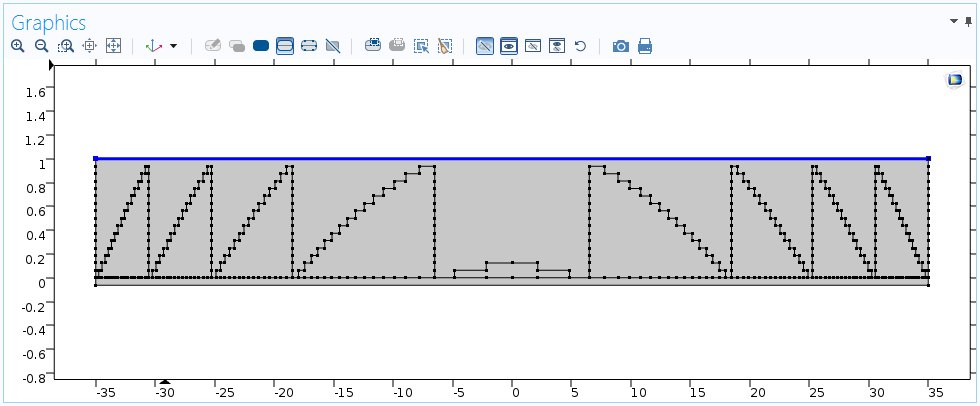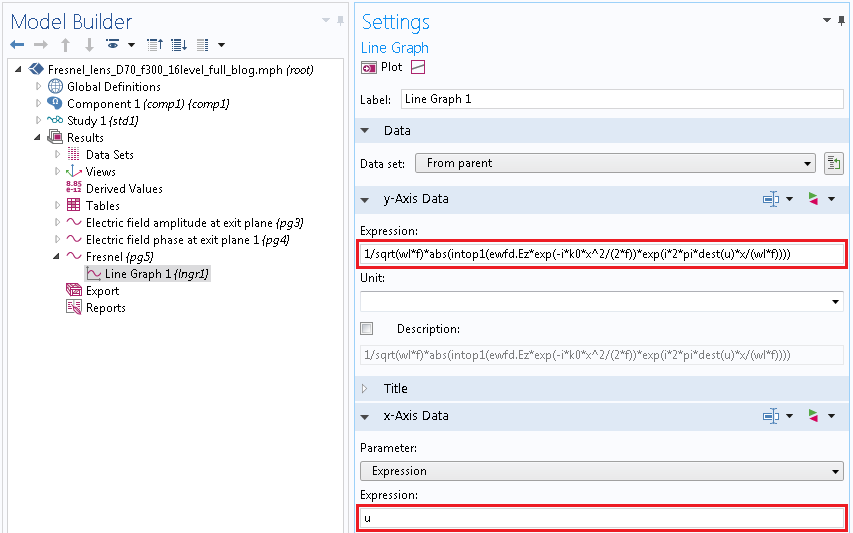COMSOL 软件中一维绘图中傅里叶变换的设置。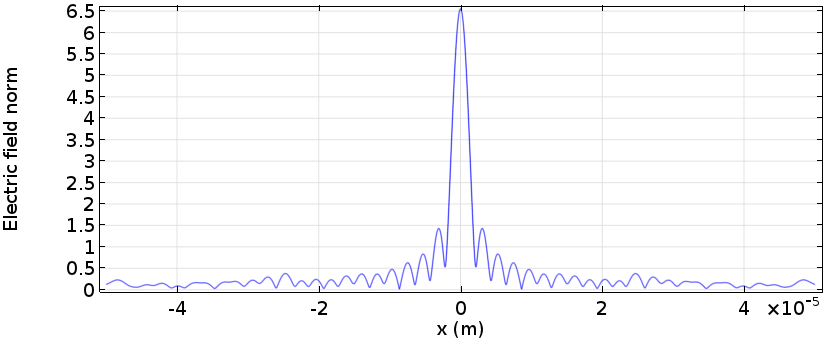### 后续操作

#### 了解有关波动光学模拟的更多信息

• J.W. Goodman, Introduction to Fourier Optics, The McGraw-Hill Company, Inc.
• D. C. O’Shea, Diffractive Optics, SPIE Press.